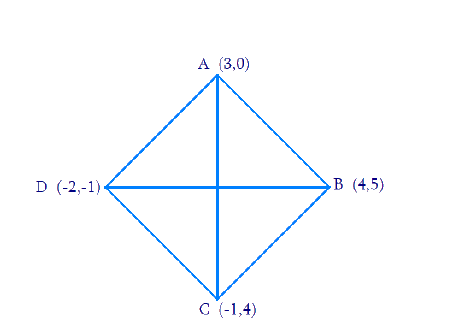# Ex.7.2 Q10 Coordinate Geometry Solution - NCERT Maths Class 10

Go back to  'Ex.7.2'

## Question

Find the area of a rhombus if its vertices are $$(3, 0)$$, $$(4, 5)$$, $$(-1, 4)$$ and $$(-2, -1)$$ taken in order.

[Hint: Area of a rhombus $$=$$ (product of its diagonals)]

Video Solution
Coordinate Geometry
Ex 7.2 | Question 10

## Text Solution

Reasoning:

A rhombus has all sides of equal length and opposite sides are parallel.

What is the known?

The $$x$$ and $$y$$ co-ordinates of the vertices of the rhombus.

What is the unknown?

The area of the rhombus

Steps:From the Figure,

Given,

• Let $$A(3, 0)$$, $$B(4, 5)$$, $$C(-1, 4)$$ and $$D(-2, -1)$$ are the vertices of a rhombus $$ABCD$$.

We know that the distance between the two points is given by the Distance Formula,

\begin{align}&\sqrt {{{\left( {{{{x}}_1} - {{{x}}_2}} \right)}^2} + {{\left( {{{{y}}_1} - {{y}_2}} \right)}^2}}\;\;\dots(1)\end{align}

Therefore, distance between $$A(3, 0)$$ and $$C (-1, 4)$$ is given by

Length of diagonal

\begin{align} AC &= \sqrt {{{[3 - ( - 1)]}^2} + {{(0 - 4)}^2}} \\ &= \sqrt {16 + 16} = 4\sqrt 2 \end{align}

Therefore, distance between $$B(4, 5)$$ and $$D(-2, -1)$$ is given by

Length of diagonal

\begin{align} BD &= \sqrt {{{[4 - ( - 2)]}^2} + \left( {5 - {{( - 1)}^2}} \right.} \\& = \sqrt {36 + 36} \\&= 6\sqrt 2 \end{align}

\begin{align} &\text{Area of the rhombus} ABCD\\ &=\! \frac{1}{2}\!\! \times\!\! \text{(Product of lengths of diagonals)} \\&= \frac{1}{2} \times {\text{AC}} \times {\text{BD}}\end{align}

Therefore, area of rhombus

\begin{align}ABCD &= \frac{1}{2} \times 4\sqrt 2 \times 6\sqrt 2 \\ &= 24 \;\rm square\; units\end{align}

Learn from the best math teachers and top your exams

• Live one on one classroom and doubt clearing
• Practice worksheets in and after class for conceptual clarity
• Personalized curriculum to keep up with school